# non-Euclidean geometry

(redirected from Non-Euclidean space)
Also found in: Dictionary, Thesaurus.

## non-Euclidean geometry,

branch of geometrygeometry
[Gr.,=earth measuring], branch of mathematics concerned with the properties of and relationships between points, lines, planes, and figures and with generalizations of these concepts.
..... Click the link for more information.
in which the fifth postulate of Euclidean geometry, which allows one and only one line parallel to a given line through a given external point, is replaced by one of two alternative postulates. Allowing two parallels through any external point, the first alternative to EuclidEuclid
, fl. 300 B.C., Greek mathematician. Little is known of his life other than the fact that he taught at Alexandria, being associated with the school that grew up there in the late 4th cent. B.C.
..... Click the link for more information.
's fifth postulate, leads to the hyperbolic geometry developed by the Russian N. I. LobachevskyLobachevsky, Nikolai Ivanovich
, 1793–1856, Russian mathematician. A pioneer in non-Euclidean geometry, he challenged Euclid's fifth postulate that one and only one line parallel to a given line can be drawn through a fixed point external to the line; he developed,
..... Click the link for more information.
in 1826 and independently by the Hungarian Janos BolyaiBolyai
, family of Hungarian mathematicians. The father, Farkas, or Wolfgang, Bolyai, 1775–1856, b. Bolya, Transylvania, was educated in Nagyszeben from 1781 to 1796 and studied in Germany during the next three years at Jena
..... Click the link for more information.
in 1832. The second alternative, which allows no parallels through any external point, leads to the elliptic geometry developed by the German Bernhard RiemannRiemann, Bernhard
(Georg Friedrich Bernhard Riemann) , 1826–66, German mathematician. He studied at the universities of Göttingen and Berlin and was professor at Göttingen from 1859.
..... Click the link for more information.
in 1854. The results of these two types of non-Euclidean geometry are identical with those of Euclidean geometry in every respect except those propositions involving parallel lines, either explicitly or implicitly (as in the theorem for the sum of the angles of a triangle).

### Hyperbolic Geometry

In hyperbolic geometry the two rays extending out in either direction from a point P and not meeting a line L are considered distinct parallels to L; among the results of this geometry is the theorem that the sum of the angles of a triangle is less than 180°. One surprising result is that there is a finite upper limit on the area of a triangle, this maximum corresponding to a triangle all of whose sides are parallel and all of whose angles are zero. Lobachevsky's geometry is called hyperbolic because a line in the hyperbolic plane has two points at infinity, just as a hyperbola has two asymptotes. The analogy used in considering this geometry involves the lines and figures drawn on a saddleshaped surface.

### Elliptic Geometry

In elliptic geometry there are no parallels to a given line L through an external point P, and the sum of the angles of a triangle is greater than 180°. Riemann's geometry is called elliptic because a line in the plane described by this geometry has no point at infinity, where parallels may intersect it, just as an ellipse has no asymptotes. An idea of the geometry on such a plane is obtained by considering the geometry on the surface of a sphere, which is a special case of an ellipsoid. The shortest distance between two points on a sphere is not a straight line but an arc of a great circle (a circle dividing the sphere exactly in half). Since any two great circles always meet (in not one but two points, on opposite sides of the sphere), no parallel lines are possible. The angles of a triangle formed by arcs of three great circles always add up to more than 180°, as can be seen by considering such a triangle on the earth's surface bounded by a portion of the equator and two meridians of longitude connecting its end points to one of the poles (the two angles at the equator are each 90°, so the amount by which the sum of the angles exceeds 180° is determined by the angle at which the meridians meet at the pole).

### Non-Euclidean Geometry and Curved Space

What distinguishes the plane of Euclidean geometry from the surface of a sphere or a saddle surface is the curvature of each (see differential geometrydifferential geometry,
branch of geometry in which the concepts of the calculus are applied to curves, surfaces, and other geometric entities. The approach in classical differential geometry involves the use of coordinate geometry (see analytic geometry; Cartesian coordinates),
..... Click the link for more information.
); the plane has zero curvature, the surface of a sphere and other surfaces described by Riemann's geometry have positive curvature, and the saddle surface and other surfaces described by Lobachevsky's geometry have negative curvature. Similarly, in three dimensions the spaces corresponding to these three types of geometry also have zero, positive, or negative curvature, respectively.

As to which of these systems is a valid description of our own three-dimensional space (or four-dimensional space-time), the choice can be made only on the basis of measurements made over very large, cosmological distances of a billion light-years or more; the differences between a Euclidean universe of zero curvature and a non-Euclidean universe of very small positive or negative curvature are too small to be detected from ordinary measurements. One interesting feature of a universe described by Riemann's geometry is that it is finite but unbounded; straight lines ultimately form closed curves, so that a ray of light could eventually return to its source.

See cosmologycosmology,
area of science that aims at a comprehensive theory of the structure and evolution of the entire physical universe. Modern Cosmological Theories
..... Click the link for more information.
; relativityrelativity,
physical theory, introduced by Albert Einstein, that discards the concept of absolute motion and instead treats only relative motion between two systems or frames of reference.
..... Click the link for more information.
.

### Bibliography

See M. J. Greenberg, Euclidean and Non-Euclidean Geometry (1980); B. A. Rosenfeld, Non-Euclidean Geometry (1988).

The Columbia Electronic Encyclopedia™ Copyright © 2013, Columbia University Press. Licensed from Columbia University Press. All rights reserved. www.cc.columbia.edu/cu/cup/
The following article is from The Great Soviet Encyclopedia (1979). It might be outdated or ideologically biased.

## Non-Euclidean Geometry

in the literal sense, any one of the geometric systems differing from Euclidean geometry. However, the term “non-Euclidean geometry” is usually applied only to geometric systems in which motion of figures has the same degree of freedom as in Euclidean geometry. The degree of freedom of motion of figures in the Euclidean plane is characterized by the fact that every figure can be moved without changing the distances between its points in such a way that any one of its points occupies any preassigned position; moreover, every figure can be rotated about any one of its points. Every figure in three-dimensional Euclidean space can be moved so that any one of its points occupies any preassigned position; in addition, every figure can be rotated about any axis passing through any one of its points.

Of particular importance among the non-Euclidean geometries are Lobachevskian geometry and Riemannian geometry. It is these geometries that one usually has in mind when one speaks of non-Euclidean geometry. Lobachevskian geometry was the first geometric system different from, and more general than, Euclidean geometry (which it includes as a limiting case). Riemannian geometry, discovered later, is in some respects the opposite of Lobachevskian geometry but nevertheless serves as its necessary complement. The comparative study of Euclidean, Lobachevskian, and Riemannian geometries has clarified their individual features, their interconnections, and their relations to other geometries. Below we compare these geometries as synthetic theories, then we discuss them in terms of differential geometry; finally, we compare their projective models.

Non-Euclidean geometries as synthetic theories. Lobachevskian geometry is based on the same axioms as Euclidean geometry, with the sole exception of the parallel postulate. According to the parallel postulate of Euclidean geometry, through a point not on a given line a there passes one and only one line coplanar with a and not intersecting a. In Lobachevskian geometry, it is assumed that there are several such lines (and then it is proved that there are infinitely many such lines).

Riemannian geometry makes use of the axiom which states that every line coplanar with a given line intersects that line. This axiom contradicts not only the parallel postulate but also other axioms of Euclidean geometry. In other words, the system of axioms underlying Riemannian geometry necessarily differs from that of Euclidean geometry in some axioms other than the parallel postulate. Thus, the axioms of order are different in these geometries: in Euclidean and Lobachevskian geometries, the order of points on a line is linear, that is, similar to the order in the set of real numbers, whereas in Riemannian geometry it is cyclic, that is, similar to the order of the points on a circle. Further, in Euclidean and Lobachevskian geometries, a line in a plane separates the plane into two parts, whereas in Riemannian geometry, a line does not separate the plane into two parts; that is, any two points in a plane not lying on a given line can be connected by a continuous arc lying in that plane and not intersecting the given line (the projective plane serves as a topological model of the Riemannian plane).

The axioms pertaining to motions of figures are identical in all three geometries. Examples of theorems in non-Euclidean geometries include the following:

(1) In Lobachevskian geometry, the sum of the interior angles of any triangle is less than two right angles. In Riemannian geometry, this sum is greater than two right angles. In Euclidean geometry, this sum is equal to two right angles.

(2) The area of a triangle in Lobachevskian geometry is given

by

(1) S = R2(π - α - β - γ)

where α, β, and γ are the interior angles of the triangle and R is a constant determined by the choice of the unit of area. In Riemannian geometry, the area is given by

(2) S = R2(α + β + γ - π)

with similar meaning for the symbols. No such relationship between the area of a triangle and the sum of its angles obtains in Euclidean geometry.

(3) There exist a number of relationships in Lobachevskian geometry between the sides and angles of a triangle, for example,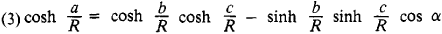where sinh and cosh are the hyperbolic sine and cosine; a, b, and c are the sides of the triangle; α, β, and γ are the angles opposite to a, b, and c; and R is a constant determined by the choice of the unit of length. For a right triangle with hypotenuse c and right angle γ, we have, for example, the equation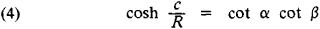The constant R in formulas (1), (3), and (4) will be the same if the unit of length bears the right relation to the unit of area. The number R is called the radius of curvature of the Lobachevskian plane (or space). For a given unit of length, the number R determines a definite segment in the Lobachevskian plane (space), which is also called the radius of curvature. If the unit of length is changed, the number R changes but the radius of curvature, as a segment, remains invariant. If the radius of curvature is taken as the unit of length, then R = 1.

Similar formulas exist in Riemannian geometry; for an arbitrary triangle,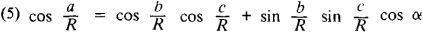and for a right triangle,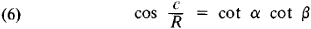where c is the hypotenuse and R is a constant. The number R is called the radius of curvature of the Riemannian plane (or space). It is evident from formulas (4) and (6) that in both non-Euclidean geometries the angles of a right triangle determine the hypotenuse. Moreover, in both non-Euclidean geometries the angles of a triangle determine the sides; that is, similar triangles are necessarily congruent. There are no formulas in Euclidean geometry similar to formulas (4) and (6) and no formulas that express linear magnitudes in terms of angles. If R is replaced by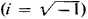, formulas (1), (3), and (4) go over

into formulas (2), (5), and (6). Quite generally, if R is replaced by Ri, all metric formulas in Lobachevskian geometry go over into the corresponding formulas in Riemannian geometry with preservation of the geometric meaning. As R tends to infinity, these formulas yield in the limit the formulas of Euclidean geometry (or lose meaning). When R tends to infinity, it means that the unit of length is infinitesimal in comparison with the radius of curvature thought of as a segment. The fact that the formulas of non-Euclidean geometry pass in the limit into the formulas of Euclidean geometry means that the relations between the elements of small (in comparison with the radius of curvature) non-Euclidean figures differ little from the relations between the elements of Euclidean figures.

Non-Euclidean geometry within the framework of differential geometry. In both non-Euclidean geometries the differential properties of the plane are similar to the differential properties of surfaces of Euclidean space (seeDIFFERENTIAL GEOMETRY). Intrinsic coordinates u, v can be introduced in a non-Euclidean plane so that the differential ds of an arc of a curve corresponding to the differentials du and dv of the coordinates is expressed by

(7) ds2 = Edu2+ 2Fdudv + Gdv2

In particular, suppose we take as the coordinate u of an arbitrary point M the signed length of the perpendicular from M to a fixed line and let the coordinate v be the signed distance from a fixed point O on this line to the base of the perpendicular. Then for the Lobachevskian plane, formula (7) will have the form

(8) ds2 = du2+ cosh2(u/R) dv2

and for the Riemannian plane,

(9) ds2 = du2+ cos2(u/R) dv2

Here, R is the radius of curvature and is identical with the constant in the previous formulas. The right-hand sides of formulas (8) and (9) are the first fundamental forms of surfaces of Euclidean space having, respectively, constant negative curvature K = —l/R2 (for example, a pseudosphere) and constant positive curvature K= 1/R2 (for example, a sphere). Therefore, the intrinsic geometry of a sufficiently small part of the Lobachevskian plane coincides with the intrinsic geometry of the corresponding part of a surface of constant negative curvature. Similarly, the intrinsic geometry of sufficiently small parts of the Riemannian plane is realized on surfaces of constant positive curvature; there exist no surfaces in Euclidean space that realize the geometry of the entire Lobachevskian plane. If R is replaced by Ri, the form (8) goes over into the form (9). Since the first fundamental form defines the intrinsic geometry of a surface, the replacement RRi takes the other metric relations of Lobachevskian geometry into metric relations of Riemannian geometry (a fact noted above). When R= ∞, both equations (8) and (9) reduce to

ds2 = du2 + dv2

that is, the first fundamental form of the Euclidean plane.

In terms of their differential properties, three-dimensional non-Euclidean spaces are Riemannian spaces in the general sense of the term. As such, they are primarily distinguished by their constant Riemannian curvature. In both the two-dimensional case and the three-dimensional case, the constancy of curvature ensures homogeneity of the space, that is, the possibility of moving figures in the space with the same degree of freedom as in the Euclidean plane and in Euclidean space, respectively. Lobachevskian space has a negative curvature —1/R2, and Riemannian space, a positive curvature l/R2 with R the radius of curvature. Euclidean space occupies an intermediate position and is a space of zero curvature.

The topological structure of spaces of constant curvature is extremely varied. In the class of spaces of constant negative curvature, Lobachevskian space is uniquely determined by two properties: it is complete (in the sense of completeness of a metric space) and it is topologically equivalent to ordinary Euclidean space. Riemannian space is uniquely determined in the class of spaces of constant positive curvature by its being topologically equivalent to projective space; n-dimensional Lobachevskian and Riemannian spaces are similarly distinguished in the class of n-dimensional spaces of constant Riemannian curvature.

Projective models of non-Euclidean geometries. Let us introduce homogeneous projective coordinates (x1,x2,x3) in the projective plane and specify some quadratic oval curve, henceforth denoted by the letter k; for example,

x12 + x22 - x32= 0

Every projective mapping of a projective plane onto itself that leaves the curve k invariant is said to be an automorphism with respect to k. Every such automorphism maps the interior points of the curve k to interior points. The set of all automorphisms with respect to k constitutes a group. Let us consider only points of the projective plane lying within k. Two figures are said to be congruent if one can be mapped onto the other by some automorphism. Since the automorphisms form a group, the following fundamental properties of congruence of figures hold: (1) if a figure A is congruent to a figure B, then B is congruent to A and (2) if A is congruent to B and B is congruent to C, then A is congruent to C. The resulting geometric system satisfies all the axioms of Euclidean geometry, except the parallel postulate, which is replaced by the Lobachevskian parallel postulate. In this way we obtain an interpretation of (two-dimensional) Lobachevskian geometry by means of objects of the projective plane or, as is usually said, a projective model of Lobachevskian geometry. The curve k is called the absolute of this model. Automorphisms with respect to k play the role of motions. Lobachevskian geometry can therefore be considered as a theory that studies the properties of figures and the quantities associated with these figures that remain invariant under automorphisms. Briefly, Lobachevskian geometry can be considered as the theory of invariants of the group of automorphisms with respect to an oval absolute.

Two-dimensional Riemannian geometry admits of a similar interpretation; it is the theory of invariants with respect to an imaginary oval absolute:

(10) x12 + x22+ x32 = 0

The points and lines of the model are all points and lines of the projective plane. The automorphisms are defined purely algebraically as the totality of linear transformations, which carry equation (10) into an equation of the same form.

Euclidean geometry can also be considered as a theory of invariants of a group of projective transformations, namely, the group of automorphisms with respect to the degenerate absolute

x12 + x22=0, x32= 0

that is, relative to the imaginary points (1, i, 0) and (1, —i, 0). These points are called circular points. The model consists of all points of the projective plane except the points on the line x3 = 0 and all lines of the projective plane except the line x3 = 0. The automorphisms play the role of similarity transformations and not of motions, as in the case of the non-Euclidean geometries.

These models represent two-dimensional geometries. Projective models of higher dimensions are analogously constructed.

In accordance with the nature of the equations of the absolutes, Lobachevskian geometry is called hyperbolic, Riemannian geometry elliptic, and Euclidean geometry parabolic.

Non-Euclidean geometries have important applications in mathematics, for example, in the theory of analytic functions and group theory, and in related fields, for example, in the theory of relativity. These applications are possible because different concrete models of non-Euclidean geometry are associated with different objects and concepts in the various branches of mathematics and in related fields.

### REFERENCES

Aleksandrov, P. S. Chto takoe neevklidova geometriia. Moscow, 1950.
Klein, F. Neevklidova geometriia. Moscow-Leningrad, 1936. (Translated from German.)
Efimov, N. V. Vysshaia geometriia, 4th ed. Moscow, 1961.

N. V. EFIMOV

The Great Soviet Encyclopedia, 3rd Edition (1970-1979). © 2010 The Gale Group, Inc. All rights reserved.
References in periodicals archive ?
Tanaka, Finite analogues of non-Euclidean spaces and Ramanujan graphs, European Journal of Combinatorics 25 (2004), 243-259.
But they could not express it in the same way, since our contemporaries must indicate their intention to exclude non-Euclidean spaces. For this reason it is misleading to regard the dethronement of Euclidean geometry as the discovery that it is false.
The examples of theory change used here, those due to the discovery of non-Euclidean spaces and the complex number system, suggest another elaboration of the Quinean view.

Site: Follow: Share:
Open / Close